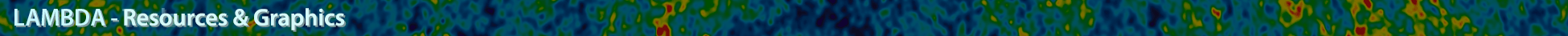# Data References

Below we document sources in the literature from which we derive the values plotted in the graphical parameter histories. Each row in the table corresponds to a data reference source. An 'x' in a parameter column means data from that row's reference was included in the parameter's graphical history plot.

Data References
Label ns t0 τ H0 Ωm Ωbh2 Ωch2 σ8 Reference
GC NGC7652 age 1996   x             Renzini et al.(1996)
COBE/DMR 1yr 1996 x               Bennett et al. (1996)
CNOC galaxy survey 1998         x       Carlberg et al. (1998)
IRAS Galaxy velocity fields 1998               x Willick and Strauss (1998)
SNIa 1999   x             Perlmutter et al. (1999)
HST_Key_Project 2001       x         Freedman et al. (2001)
D/H+BBN 2001           x     Pettini and Bowen (2001)
White dwarf dating 2002   x             Hansen et al. (2002);
Richer et al. (2002)
Galaxy bias + z distort 2002         x       Verde et al. (2002)
Clusters+SNIa 2002           x     Steigman et al. (2002)
Boomerang 2002a x x x     x x   Netterfield et al. (2002)
DASI+DMR 2002 x         x x   Pryke et al. (2002)
CBI SZ 2002               x Komatsu and Seljak (2002)
CBI+DMR 2003           x     Sievers et al. (2003)
D/H+BBN 2003           x     Kirkman et al. (2003)
X-ray cluster abunduance 2003               x Bahcall and Bode (2003)
WMAP1++ 2003b     x           Spergel et al. (2003)
SNIa 2004         x       Riess et al. (2004)
CHANDRA+tSZ 2006       x         Bonamente et al. (2006)
Cepheids+SNIa 2011       x         Riess et al. (2011)
D/H+BBN 2012           x     Pettini and Cooke (2012)
WMAP9++ 2013c x x x x x x x   Hinshaw et al. (2013)
WMAP9 2013       x         Hinshaw et al. (2013)
BOSS Lyα forest 2013 x             x Palanque-Delabrouille et al. (2013)
BAO+LSS 2013               x Addison et al. (2013)
SPT+tau_prior 2013       x         Story et al. (2013)
Planck SZ+BAO+BBN         x     x Planck Collaboration (2014)
SPT SZ bispectrum 2014               x Crawford et al. (2014)
ACT SZ PDF 2014               x Hill et al. (2014)
BOSS weak lensing 2015         x     x More et al. (2015)
SNIa 2014         x       Betoule et al. (2014)
Planck_PR2++ 2015d     x           Planck XIII (2015)
Inv_Dist_Ladder 2015       x         Aubourg et al. (2015)
D/H+BBN 2016           x     Cooke et al. (2016)
ACTPol 2017       x         Louis et al. (2017)
GravLens_Time_Delay 2019       x         Wong et al. 2019
SPTPol 2017       x         Henning et al. (2017)
Planck-PR3++ 2018e x x x x x x x x Planck VI (2018)
Pantheon_SNIa 2018         x       Scolnic et al. (2018)
Planck-PR3 2018       x         Planck VI (2018)
XMM+Planck_tSZ 2018       x         Kozmanyan et al. (2018)
TRGB Dist Ladder       x         Freedman et al. (2019)
Megamasers 2020       x         Pesce et al. (2020)
eBOSS_BBN+BAO 2020       x         eBOSS Collaboration (2020)
ACTPol_DR4       x         Aiola et al. (2020)
SPT-3G_TE+EE       x         Dutcher et al. (2021)
SBF_distances 2021       x         Blakeslee et al. (2021)
LIGO-Virgo-KAGRA Collaboration (2021)       x         LIGO-Virgo-KAGRA Collaboration (2021)
Cepheids+SNIa 2021       x         Riess et al. (2021)
Planck_PR3+S2 2020     x           Pagano et al. (2020)
Planck_PR4 2020     x           Planck Int. LVII (2020)
Planck_PR4 2023     x           Tristram et al. (2023)

aBoomerang τ value is an upper limit prior.

bDerived from multiple data sources: WMAP+ACBAR+CBI+2dFGRS+Lyα

cDerived from multiple data sources: WMAP+eCMB+BAO+Ho

dDerived from multiple data sources: TT+TE+EE+lowP+lens+ext.

e Derived from multiple data sources: TT+TE+EE+lowE+lens+BAO

Contributed by the NASA / LAMBDA Archive Team.

A service of the HEASARC and of the Astrophysics Science Division at NASA/GSFCHEASARC Director: Dr. Andrew F. Ptak
LAMBDA Director: Dr. Thomas M. Essinger-Hileman
NASA Official: Dr. Thomas M. Essinger-Hileman
Web Curator: Mr. Michael R. Greason